[ Home > Magnetic field from a 1m x 1m square radiating coil ]

# Magnetic field from a 1m x 1m swuare radiating coil

Note: these graphs show calculated Z-axis (perpendicular to the radiating coil in X-Y plane) components of magnetic field strength, relative to the value at the center of the coil.

## In a plane parallel to the radiating coil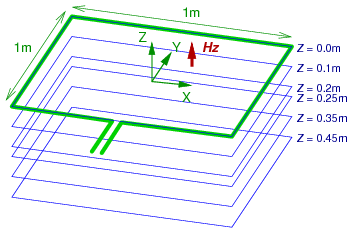### Z = 0m### Z = 0.1m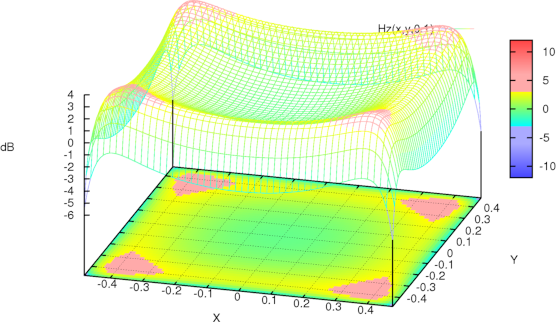### Z = 0.2m### Z = 0.25m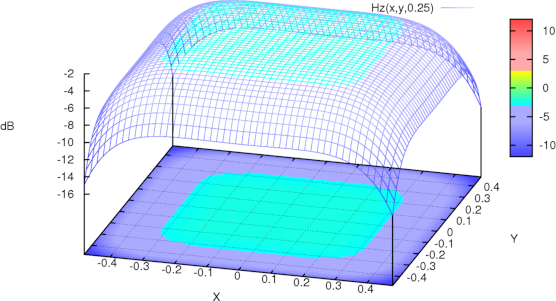### Z = 0.35m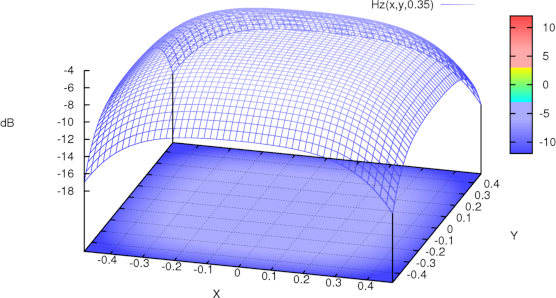### Z = 0.45m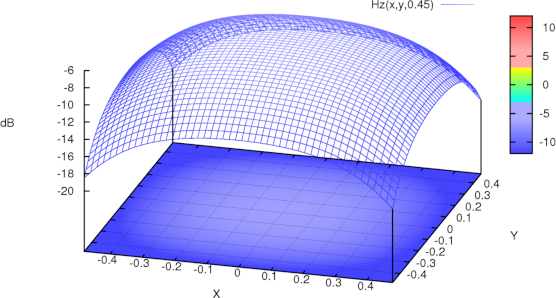## In a plane perpendicular to the radiating coil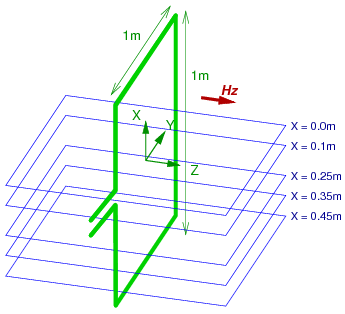### X = 0m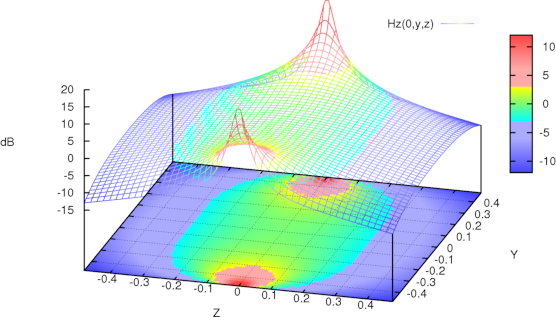### X = 0.1m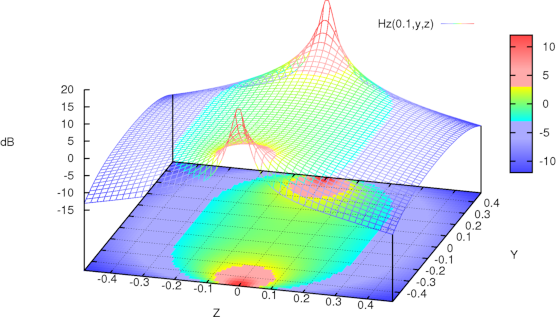### X = 0.25m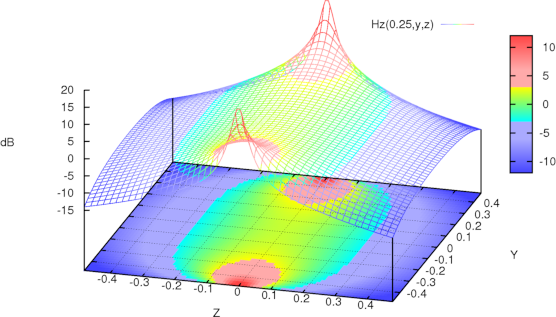### X = 0.35m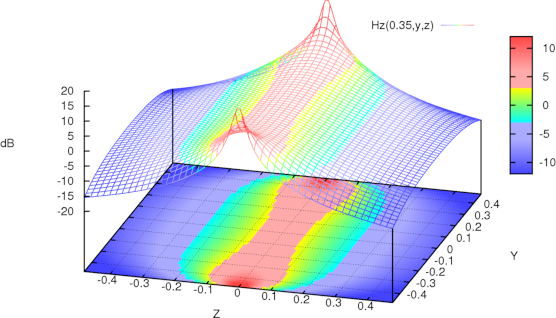### X = 0.45m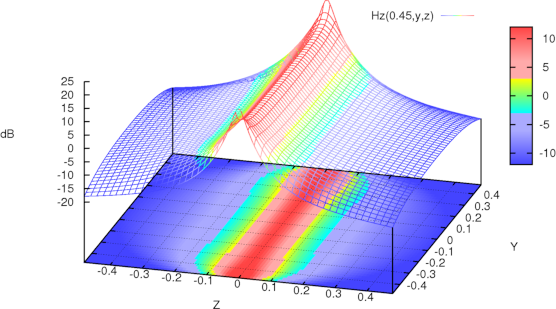### X = 0m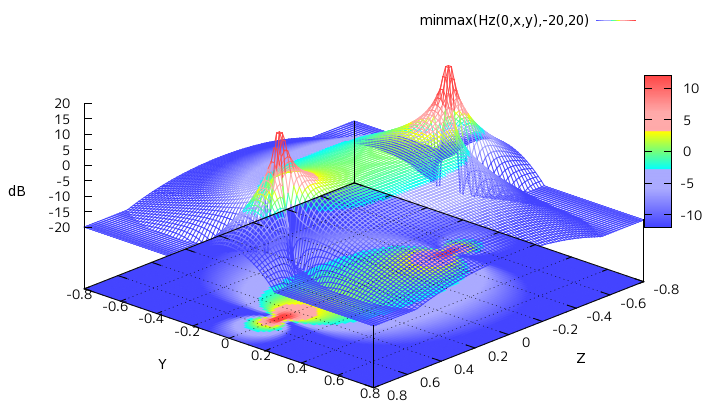## Magnetic field strength at center of rectangular coil

Magnetic field strength at center of rectangular coil of w × h (m) may be calculated with: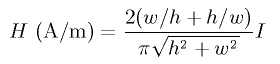For square coil of w × w (m), it may be simplfied to: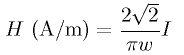## Inductance of of rectangular coil

Inducatence of rectangular coil of w × h (m), made from conductor with radius r can be estimated with: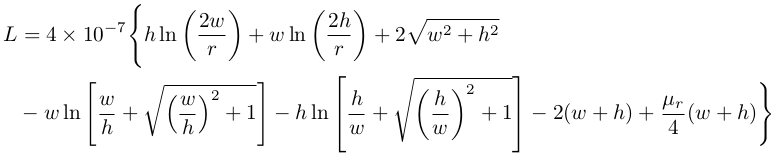For square coil of w × w (m), it may be simplfied to: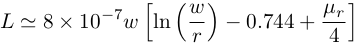## Reference

• Equations for the Magnetic Field Produced by One or More Rectangular Loop, Martin Misakian, Journal of Research of the National Institute of Standards and Technology, Volume 105, Number 4, July–August 2000
• Electromagnetic Compatibility Handbook, Kenneth L. Kaiser, 2004
• IEC 61000-4-8, Electromagnetic Compatibility (EMC) - Part 4-8: Testing and Measurement Techniques - Power Frequency Magnetic Field Immunity Test, IEC

2012-05-05, T.Sato